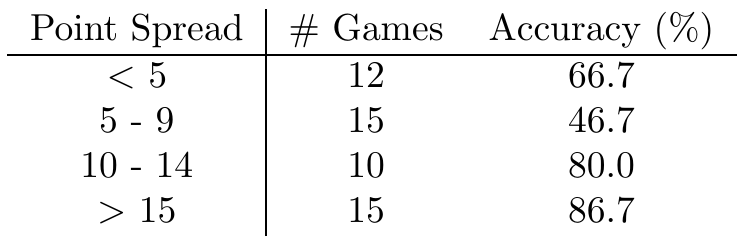Another pretty good result this week: $36/52 = 69.2%$. Accuracy by spread is at right.
A quick note on accuracy as an evaluation metric: We actually predict significant fluctuations in performance of our model from week to week. If we estimate our prediction accuracy at 70%, then for a week with $n\approx 50$ games we expect a distribution of accuracies from week to week with standard deviation given by the binomial distribution: $\sigma = \sqrt{n p (1-p)}/n \approx 6.5\%$. This is a rather broad distribution – we might expect results ranging from $60\%$ to $80\%$ to be quite common from week to week. For $n \approx 15$ games (as in each row of our tables comparing by point spread), the standard deviation increases by a factor of $\approx 2$.